SYLLABUS  Previous: 3 FINITE ELEMENT METHOD  Up: 3 FINITE ELEMENT METHOD  Next: 3.2 An engineer's formulation

## 3.1 Mathematical background

Slide : [ Weighted residuals - Variational form - Partial integration - Approximation - BC || VIDEO login]

To approximate a set of linear partial differential equations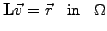(1)

for an unknown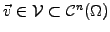that is continuously defined with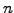derivatives in the volume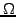and is subject to the boundary conditions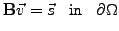(2)

a mathematician involves first the so-calledWeighted residuals.
Having defined a scalar product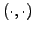and a norm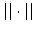, the calculation essentially amounts to the minimization of a residual vector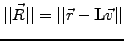(3)

which is carried out using tools from the variational calculus.Variational principle.
A quadratic form is constructed for that purpose by choosing a test function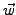in a sub-space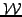that is sufficiently general'' and satisfies the boundary conditions. The linear equation (3.1#eq.1) can then be written as an equivalent variational problem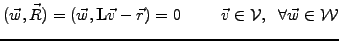(4)Integration by parts.
Ifis differentiable at least once in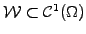, the regularity required by the forth-coming discretization can often be relaxed by partial integrations. Using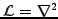for illustration, Leibniz' rule states that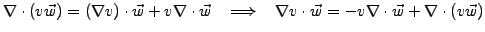(5)

Integrating over the volumeand using Gauss' divergence theorem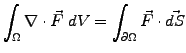yields a generalized formula for partial integration: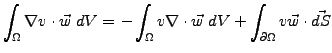(6)

For the special case where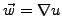, this is known as Green's formula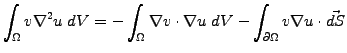(7)

The last (surface-) term can sometimes be imposed to zero (or to a finite value) when applying so-called natural boundary conditions.Numerical approximation.
It turns out that the formulation as a variational problem is general enough that the solution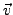of (3.1#eq.4) remains a converging approximation of (3.1#eq.1) even when the sub-spaces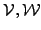are restricted to finite, a priori non-orthogonal, but still complete sets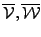of functions. The overlap integrals involving non-orthogonal functions are often evaluated with a numerical quadrature that can be easily be handled by the computer.

In general, the discretized solutionis expanded in basis functions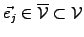, which either reflect a property of the solution (e.g. the operator Green's function in the Method of Moments), or which are simple and localized enough so that they yields cheap inner products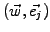and sparse linear systems (e.g. the roof-top function for the linear Finite Element Method). Different choices can also be made for the test functions: among the most popular are the Galerkin method, where test and basis functions are both chosen from the same sub-space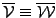and the method of collocation, which consists in taking Dirac functions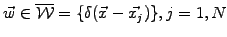that lead to point-wise evaluations of the integrand on the mesh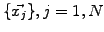.Boundary conditions.
If natural boundary conditions are not already sufficient, essential boundary conditions have to be imposed either by choosing the functional space(e.g. let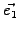and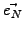overlap in a periodic domain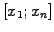as in the next section) or by replacing one or several equations of the linear system (e.g. use higher order finite differences to preserve the convergence rate as in exercise 3.02).

SYLLABUS  Previous: 3 FINITE ELEMENT METHOD  Up: 3 FINITE ELEMENT METHOD  Next: 3.2 An engineer's formulation